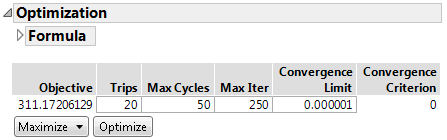Profilers > Custom Profiler > The Custom Profiler Report > Optimization Settings and Controls
Publication date: 05/24/2021

## Optimization Settings and Controls

Figure 7.7 Optimization Settings and ControlsFormula

The formula to be optimized. When a single response is used, the expression is the response column name. When multiple responses are used, the expression is a sum of desirability functions. You can edit the objective expression.

Objective

The current value of the objective function. When a single response is used, the objective expression is the predicted response. When multiple responses are used, the objective expression is the desirability function. For more information about desirability functions, see Desirability Profiling and Optimization.

Trips

The number of random starts in the optimization algorithm. Each trip restarts the algorithm at a different starting point. This guards against finding local solutions.

Max Cycles

The maximum number of cycles used in the optimization algorithm. Each cycle is single pass through the input parameters and optimizes each one individually.

Max Iter

The maximum number of optimization iterations per cycle for each input parameter.

Convergence Limit

The upper limit for the convergence criterion for the optimization algorithm. If the convergence criterion becomes less than this value, the algorithm stops.

Convergence Criterion

The value of the convergence criterion for the optimization algorithm.

Maximize

Enables you to choose to maximize or minimize the objective function.

Optimize

Starts the optimization algorithm.